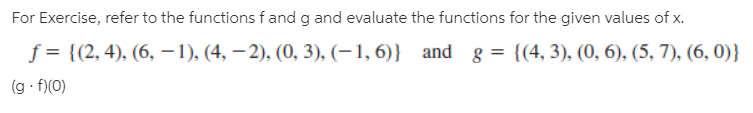# For Exercise, refer to the functions f and g and evaluate the functions for the given values of x. f = {(2, 4), (6, -1), (4, –2), (0, 3), (–1, 6)} and (g f)(0) g = {(4, 3), (0, 6), (5, 7), (6, 0)}

Questionhelp_outlineImage TranscriptioncloseFor Exercise, refer to the functions f and g and evaluate the functions for the given values of x. f = {(2, 4), (6, -1), (4, –2), (0, 3), (–1, 6)} and (g f)(0) g = {(4, 3), (0, 6), (5, 7), (6, 0)} fullscreen